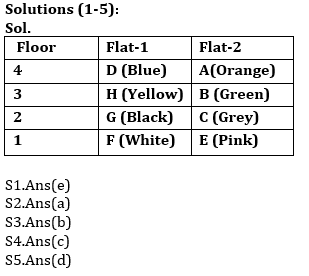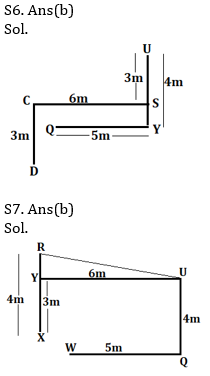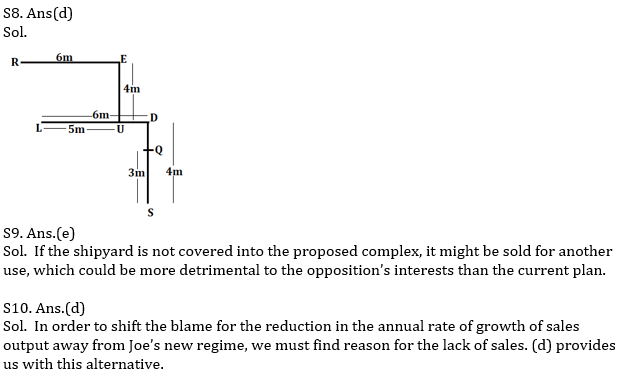Latest Banking jobs   »   Reasoning Ability Quiz For SBI/IBPS PO...

Reasoning Ability Quiz For SBI/IBPS PO Mains 2021- 11th December

Directions (1-5): Study the information carefully and answer the questions given below.
Eight persons A, B, C, D, E, F, G and H are living on four different floors of a building. Ground floor is numbered 1 and top floor is numbered four. There are two flats on each floor- flat-1 and flat-2 from west to east such that flat 1 is west of flat 2. Flat-1 of second floor is exactly above flat-1 of first floor and other flats are placed in the same way. All of them likes different colours i.e. Blue, black, green, Orange, Yellow, Pink, Grey and white but not necessary in same order.
A likes orange and lives in even number flat. D lives North West of E’s flat. C lives one of the floor below D’s floor. One floor is between H and F, who likes white colour. H and F lives on same flat number. E does not live on second floor. Only one floor gap between the one who likes grey colour and the one who likes blue colour. The one who likes Black colour does not live in even number flat. B lives east of H who lives south of D. G does not live in the same flat number in which E lives. G lives above E. the one who likes Yellow lives west of the one who likes Green colour. The one who likes blue colour lives above the floor of one who likes Yellow colour. The one who likes Pink colour lives in even number flat.

Q1. Who among the following person lives immediate above flat of C?
(a) A
(b) D
(c) H
(d) F
(e) None of these

Q2.Who among the following person lives in flat 2 of floor 4?
(a) A
(b) C
(c) D
(d) H
(e) None of these

Q3.Who among the following person lives South west of B and North west of E?
(a) B
(b) G
(c) C
(d) E
(e) None of these

Q4. How many floor between D and the one who likes pink colour?
(a) One
(b) Three
(c) Two
(d) None
(e) Both Live at same floor

Q5 Who among the following person likes grey colour?
(a) B
(b) F
(c) H
(d) C
(e) None of these

Directions (6-8): Each of these questions is based on the following information:
(i) A * B means A is 5m west of B.
(ii) A \$ B means A is 6m east of B.
(iii) A % B means A is 3m north of B.
(iv) A @ B means A is 4m south of B.

Q6. If the expression ‘Q*Y@U%S\$C%D’ is true, what is the direction of Y with respect to C?
(a) North
(b) South-East
(c) South-West
(d) North-East
(e) None of these

Q7. If the expression ‘W*Q@U\$Y%X@R’ is true, What is the Shortest distance between R and U?
(a) 6m
(b) √37m
(c) √35m
(d) √41m
(e) 8m

Q8. If the expression ‘Q%S@D\$L*U@E\$R’ is true, what is the direction of E with respect to Q?
(a) South- West
(b) North-East
(c) South- East
(d) North- West
(e) East

Q9. Prompted by a proposal to convert a shipyard into a complex of condominiums with a full-service marina and boat repair centre and by concern about the proposal from local residents, baymen, and environmentalists, the town is considering a one-year building moratorium for the waterfront area.
Which of the following, if true, would most seriously weaken opposition to the complex?
(a) Condominiums would sell for \$350,000 each.
(b) There is large demand for boat repair services.
(c) A growing population results in the closure of shellfish.
(d) There are already 1200 moorings on the waterfront.
(e) The shipyard may be sold for another commercial use.

Q10. Before the arrival of Joe, a new partner, sales output in Bill’s company, Midas in Revere Ltd., had been rising by 10% per year on average. Innovations by Joe included computerization of technical processes and reductions in the work force, but annual sales output has only risen by 5% per year. It appears that Joe’s innovations have caused the reduction in the annual growth rate.
Which of the following, if true, would most seriously weaken the conclusions above?
(a) The investment in new machinery entails a provision for depreciation of the cost of the fixed assets, which causes a reduction in profit.
(b) Midas in Reverse Ltd. Does not base increase in the selling price of its products with costs.
(c) Joe’s innovations were intended as long-term investment and not made for short-term profit growth.
(d) General demand for the product manufactured by the company has declined.
(e) Workers laid off by Midas in Reverse Ltd. have been hired by a competitor who is taking an increasing share of the market.

Directions (11-15): There are two rows given and to find out the resultant of a particular row we need to follow the following steps: –
Step 1: If an even number is followed by an odd number then the resultant will be the addition of both the numbers.
Step 2: If an odd number is followed by a perfect square then the resultant will be the difference of that square number and the odd number.
Step 3: If an odd number is followed by another odd number (but not a perfect square) then the resultant will be the addition of both the numbers.
Step 4: If an odd number is followed by an even number (but not a perfect square) then the resultant comes by multiplying the numbers.
Step 5: If an even number is followed by another even number then the resultant will be the division of first number by the second number.

Q11. Find the sum of two rows
20     4      5
17     12    11

(a) 178
(b) 205
(c) 223
(d) 225
(e) None of these

Q12. If the sum of the resultants of two rows is 18. Then find the value of X.
21    9    6
50    2    X

(a) 16
(b) 12
(c) 9
(d) 15
(e) None of these

Q13. Find the difference between the resultant of first and second row.
25    7    16
8    17    12

(a) 300
(b) 298
(c) 302
(d) 287
(e) None of these

Q14. Find the multiplication of the resultant of first and second row.
51    21    4
16    17    5

(a) 610
(b) 684
(c) 722
(d) 720
(e) None of these

Q15. Find the sum of the resultant of first and second row.
7    8    13
5    16   9

(a) 69
(b) 71
(c) 68
(d) 73
(e) None of these

SolutionsS11. Ans(d)
Sol. For row-1:
An even number is followed by another even number = 20/4 = 5
An odd number is followed by another odd number (but not a perfect square) = 5 + 5 = 10
For row-2:
An odd number is followed by an even number (but not a perfect square) = 17×12 =204
An even number is followed by an odd number = 204 + 11 = 215
The sum is= 215+10=225

S12. Ans(c)
Sol. For row -1:
An odd number is followed by a perfect square = 21-9 = 12
An even number is followed by a even number = 12/6 = 2
For row – 2:
An even number is followed by another even number = 50/2 = 25
The resultant of row-2 is = 18-2 = 16
Then value of X must be = 9

S13. Ans(b)
Sol. For row – 1:
An odd number is followed by another odd number (but not a perfect square) = 25 + 7 = 32
An even number is followed by an even number = 32/16 = 2
For row – 2:
An even number is followed by an odd number = 8 + 17 = 25
An odd number is followed by an even number (but not a perfect square) = 25×12 = 300
The difference of row-1 and row-2= 300 – 2 =298

S14. Ans(b)
Sol. For row – 1:
An odd number is followed by another odd number (but not a perfect square) = 51 + 21= 72
An even number is followed by another even number = 72/4 = 18
For row – 2:
An even number is followed by an odd number = 16 + 17 = 33
An odd number is followed by another odd number (but not a perfect square) = 33 + 5 = 38
The multiplication of row-1 and row-2= 18 x 38 = 684

S15. Ans(b)
Sol. For row – 1:
An odd number is followed by an even number (but not a perfect square) = 7 x 8 = 56
An even number is followed by an odd number = 56 + 13 = 69
For row – 2:
An odd number is followed by a perfect square =16-5 = 11
An odd number is followed by a perfect square =11 – 9 = 2
The multiplication of row-1 and row-2= 69 + 2 = 71×

Thank You, Your details have been submitted we will get back to you.Join India's largest learning destination

What You Will get ?

•Job Alerts
•Daily Quizzes
•Subject-Wise Quizzes
•Current Affairs
•Previous year question papers
•Doubt Solving session

ORJoin India's largest learning destination

What You Will get ?

•Job Alerts
•Daily Quizzes
•Subject-Wise Quizzes
•Current Affairs
•Previous year question papers
•Doubt Solving session

ORJoin India's largest learning destination

What You Will get ?

•Job Alerts
•Daily Quizzes
•Subject-Wise Quizzes
•Current Affairs
•Previous year question papers
•Doubt Solving session

Enter the email address associated with your account, and we'll email you an OTP to verify it's you.Join India's largest learning destination

What You Will get ?

•Job Alerts
•Daily Quizzes
•Subject-Wise Quizzes
•Current Affairs
•Previous year question papers
•Doubt Solving session

Enter OTP

Please enter the OTP sent to
/6

Did not recive OTP?

Resend in 60sJoin India's largest learning destination

What You Will get ?

•Job Alerts
•Daily Quizzes
•Subject-Wise Quizzes
•Current Affairs
•Previous year question papers
•Doubt Solving sessionJoin India's largest learning destination

What You Will get ?

•Job Alerts
•Daily Quizzes
•Subject-Wise Quizzes
•Current Affairs
•Previous year question papers
•Doubt Solving session

Almost there

+91Join India's largest learning destination

What You Will get ?

•Job Alerts
•Daily Quizzes
•Subject-Wise Quizzes
•Current Affairs
•Previous year question papers
•Doubt Solving session

Enter OTP

Please enter the OTP sent to Edit Number

Did not recive OTP?

Resend 60

By skipping this step you will not recieve any free content avalaible on adda247, also you will miss onto notification and job alerts

Are you sure you want to skip this step?

By skipping this step you will not recieve any free content avalaible on adda247, also you will miss onto notification and job alerts

Are you sure you want to skip this step?